Search

About 47 Search Results Matching Types of Worksheet, Worksheet Section, Generator, Generator Section, Subjects matching Graphing, Similar to Reading a Protractor Worksheet 2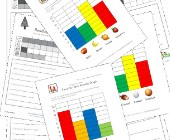Reading a Graph Worksheets

This set of graphing worksheets asks kids to look ...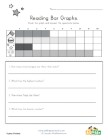Patriotic Bar Graph Worksheet

Use the bar graph to answer the questions about th...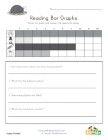Thanksgiving Bar Graph Worksheet

Use the bar graph to answer the questions about Th...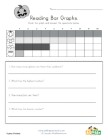Halloween Bar Graph Worksheet

Use the bar graph to answer the questions about Ha...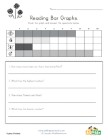Spring Bar Graph Worksheet

Use the bar graph to answer the questions about th...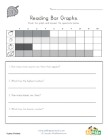Fall Bar Graph Worksheet

Use the bar graph to answer the questions about th...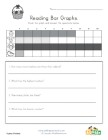Easter Bar Graph Worksheet

Use the bar graph to answer the questions about Ea...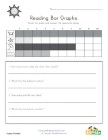Summer Bar Graph Worksheet

Use the bar graph to answer the questions about th...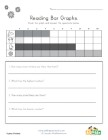Winter Bar Graph Worksheet

Use the bar graph to answer the questions about th...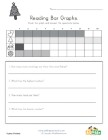Christmas Bar Graph Worksheet

Use the bar graph to answer the questions about Ch...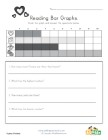Valentine's Day Bar Graph Worksheet

Use the bar graph to answer the questions about Va...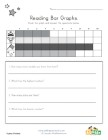Memorial Day Bar Graph Worksheet

Use the bar graph to answer the questions about Me...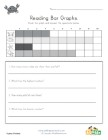Ocean Themed Bar Graph Worksheet

Use the bar graph to answer the questions about oc...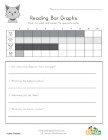Animal Themed Bar Graph Worksheet

Use the bar graph to answer the questions about an...